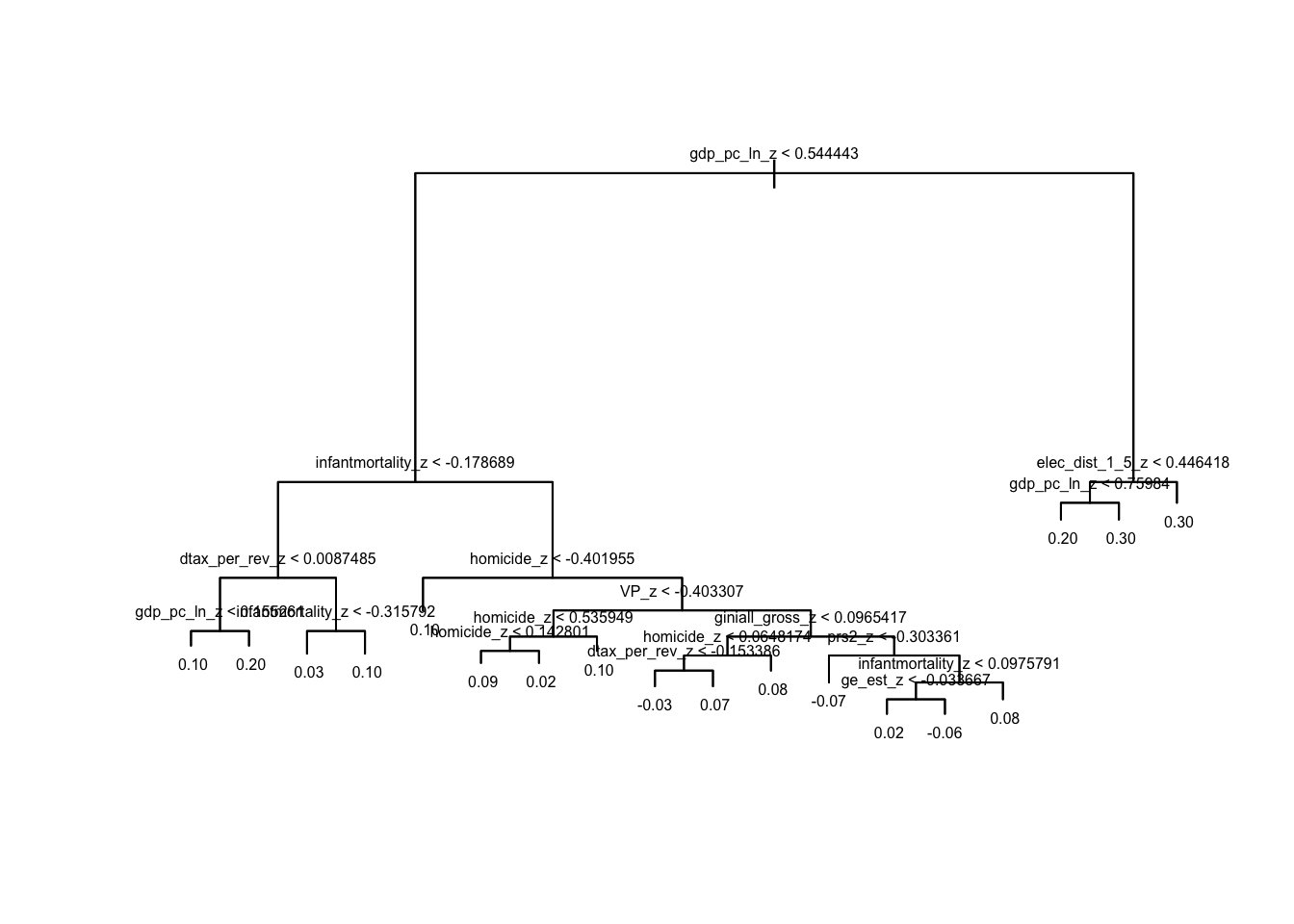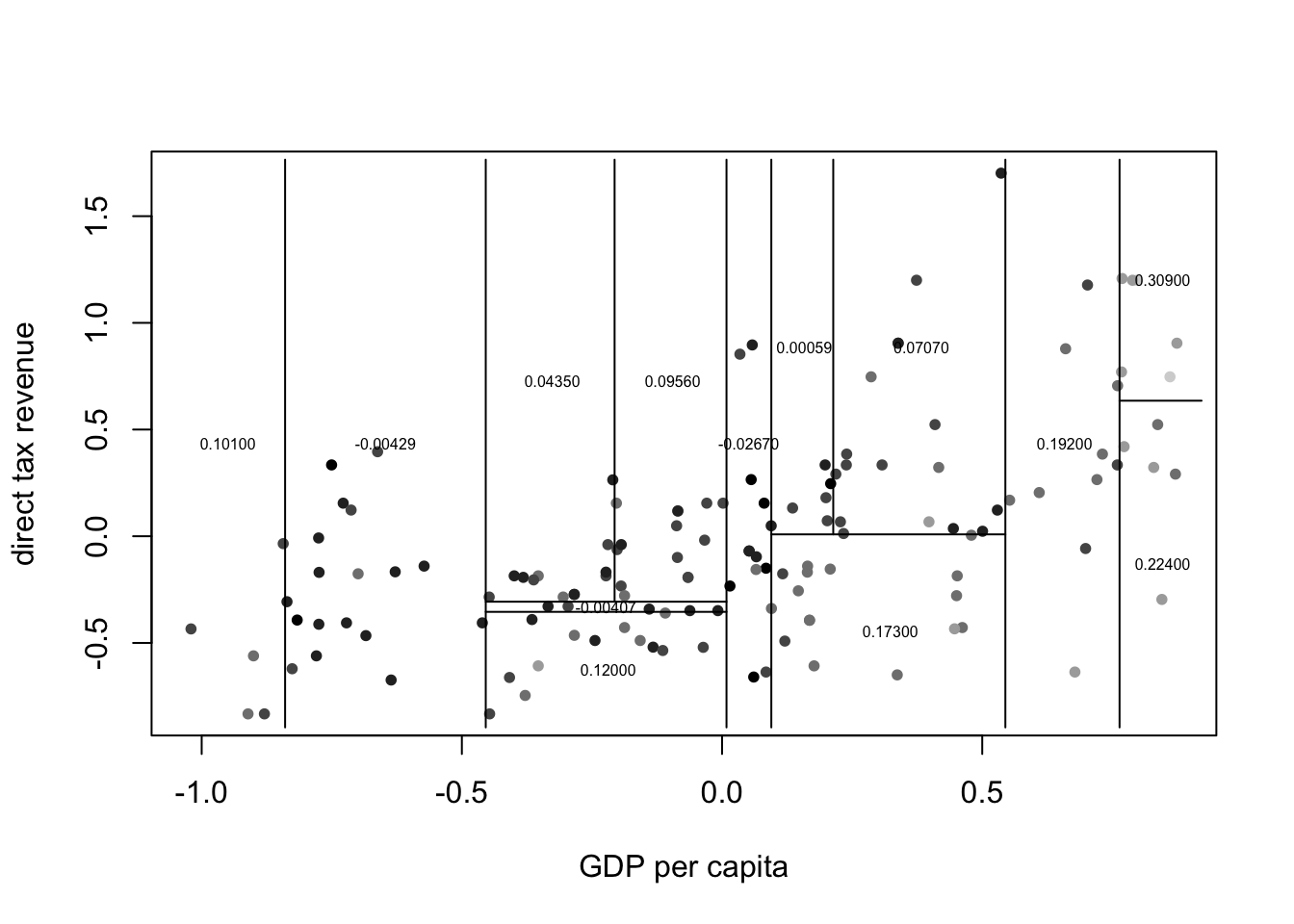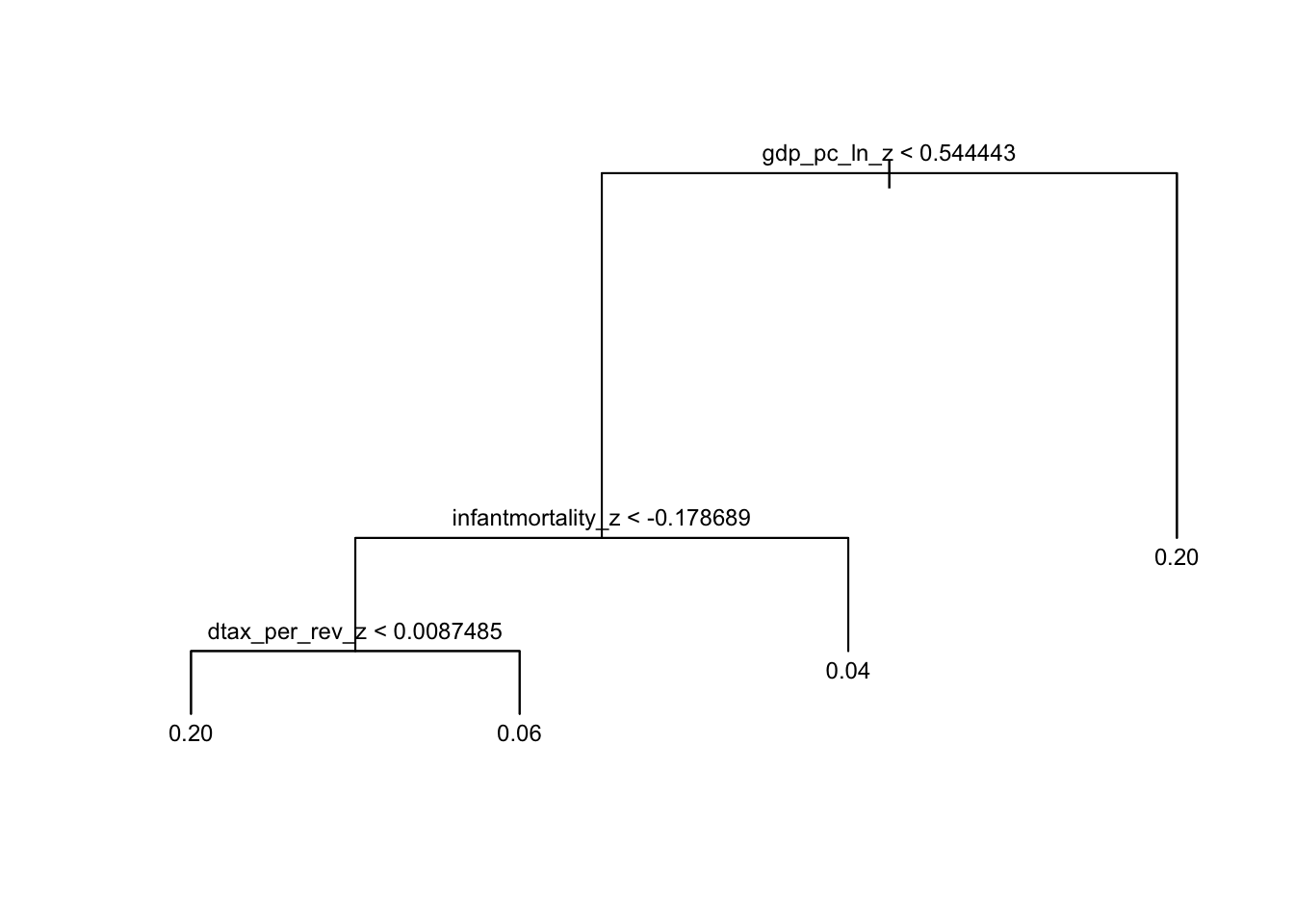Let’s apply these techniques to a subject that I know nothing about: differences in voting by economic status across the world. Read in the datafile votes.RData, which is a subset of the data contained in Kasara and Suryanarayan (2015).1 These data2 provide an excellent illustration of some of the benefits of tree methods. Due to their small sample size of 211, the authors have to run 6 separate regressions to test each of their proposed explanations in turn. With a tree-based approach, we can include all 6 explanatory variables, and controls, in a single analysis and explore how much explanatory power each contributes.

library(reshape2) # reshaping data for plots
library(ggplot2) # plots

# source custom ggplot theme
source('https://raw.githubusercontent.com/jayrobwilliams/RWmisc/master/theme_rw.R')

# load data
load('votes.RData')

# split into test and training data
train <- sample(1:nrow(votes), (2 / 3) * nrow(votes))
votes_train <- votes[train, ]
votes_test <- votes[-train, ]

The first variable in the data is the estimated $$\beta_j$$ effect of income on turnout. The next six are the theoretically driven explanatory variables: voting polarization, electoral distance between the top and bottom income quintiles, bureaucratic quality, government effectiveness, direct tax revenue, and log GDP per capita. The remaining variables are the controls: proportional representation, concurrent elections, compulsory voting, polity score, infant mortality, gross gini coefficient, homicide rate, and ethnic fractionalization.

All of these methods select which predictors to include and which to exclude in a model. Some of them even allow for $$n$$-way interactions and $$n^{th}$$ order polynomials. This can result in very complex models where it is difficult to recover the marginal effect of a specific variable. If we were using synethic data and controlled the data generating process, we could compare the choices made by these models to true DGP. When working with real data, we don’t have this option. We thus rely on predictive accuracy as a measure of model fit. Many packages use mean squared error (MSE) as their metric of fit. \begin{align} \frac{1}{n}\sum_{i=1}^{n} \left(\hat{Y_i} - Y_i \right)^2 \end{align} Some packages don’t directly provide a MSE, but they all allow us to calculate predicted $$\hat{y}$$s from our test data, and we can then compute MSE ourselves. Let’s go ahead and make a function to calculate MSE because we’re going to be doing this a lot.

# calculate mean squared error
mse <- function(y, yhat) {

mean((yhat - y)^2)

}

## Single Tree Approaches

The tree package is a little outdated and doesn’t have a lot of options compared to newer packages like rpart, but it does offer some really easy plotting functions to help us understand how regression trees work.

library(tree)
fit_tree <- tree(formula = beta_exp ~., data = votes_train)
summary(fit_tree)
##
## Regression tree:
## tree(formula = beta_exp ~ ., data = votes_train)
## Variables actually used in tree construction:
##  "gdp_pc_ln_z"       "infantmortality_z" "dtax_per_rev_z"
##  "homicide_z"        "VP_z"              "giniall_gross_z"
##  "prs2_z"            "ge_est_z"          "elec_dist_1_5_z"
## Number of terminal nodes:  18
## Residual mean deviance:  0.0043 = 0.52 / 122
## Distribution of residuals:
##    Min. 1st Qu.  Median    Mean 3rd Qu.    Max.
##  -0.132  -0.041  -0.004   0.000   0.042   0.167

This is a relatively complex tree that only uses six out of 14 variables to make classification choices. Let’s visually inspect the tree to see which variables are doing most of the heavy lifting in sorting outcomes. Use the plot() and text() commands on our model object to get a visual version of this decision tree. The text() command is finnicky, so make sure you execute it in the same command as plot().

# need to execute these as part of the same command
plot(fit_tree); text(fit_tree, cex = .5)This plot tells us a couple things, the most important of which is that this model will only ever predict a small number of different $$beta_j$$ values, even though we have 211 unique values in the data. The plot shows multiple leaves with a value of 0.10. This is due to rounding; the actual values are different. Access the frame within our tree fit object. The yval column for any row that is a <leaf> is a predicted value that our model can produce. See for yourself that we actually have eight different predicted values, rather than the seven that the plot shows.

fit_tree$frame Before we get into more advanced methods, let’s spend a little more time with tree() and use it to get a better idea of how this classification works. Fit a new regression tree that only uses GDP per capita and direct tax revenue (the two predictors after the initial split in our tree). Plot these two variables against each other, with the color of the points reflecting the estimated effect of income on turnout (the grey() and findInterval() functions will be helpful here, if you don’t want to have to use colorRampPalette()). Then use the partition.tree() command on our tree fit object, and be sure to set add = T to overlay the partitions. partition.tree() is also finnicky, so make sure it’s executed in the same command as plot(). fit_tree_simple <- tree(formula = beta_exp ~ gdp_pc_ln_z + dtax_per_rev_z, data = votes_train) plot(x = votes_train$gdp_pc_ln_z, y = votes_train$dtax_per_rev_z, col = grey(seq(0, 1, length.out = 7))[findInterval(votes_train$beta_exp,
seq(min(votes_train$beta_exp), max(votes_train$beta_exp),
length.out = 7))], pch = 20,
xlab = 'GDP per capita', ylab = 'direct tax revenue')
partition.tree(fit_tree_simple, add = T, cex = .5)We can see where each of the countries in the training dataset fall along the two variables used in our decision tree, and each square represents a leaf at the bottom of the tree. The colors range from lightest to darkest, covering the range of $$\beta_j$$ in the data.

The last thing we want to do with tree() is see how changing the parameters of the tree algorithm can alter the resulting model. The decision of whether to grow a new node or not is controlled by a number of parameters, but let’s focus on the mindev argument. This determines how much a potential node must reduce the error for the algorithm to grow a new node. The default value is .01. Try setting it to something larger and plotting the resulting tree.

fit_tree <- tree(beta_exp ~., data = votes_train, mindev = .05)
plot(fit_tree); text(fit_tree, cex = .75)Our tree is now more simpler and makes splits based only on GDP per